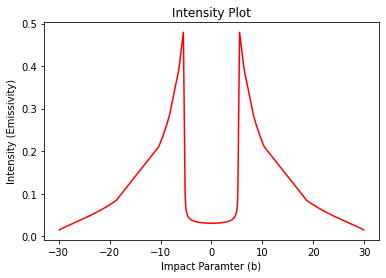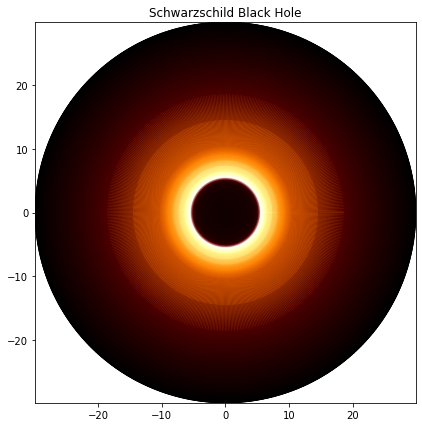# Shadow cast by an thin emission disk around a Schwarzschild Black Hole¶

In :
import astropy.units as u



Defining the conditions

In :
mass = 1 * u.kg
fov = 30 * u.km
# What field of view is the user expecting

In :
shadow = Shadow(mass=mass, fov=fov, n_rays=1000)

In :
obj = ShadowPlotter(shadow=shadow, is_line_plot=True)
obj.plot()
obj.show()In :
obj = ShadowPlotter(shadow=shadow, is_line_plot=False)
obj.plot()
obj.show()